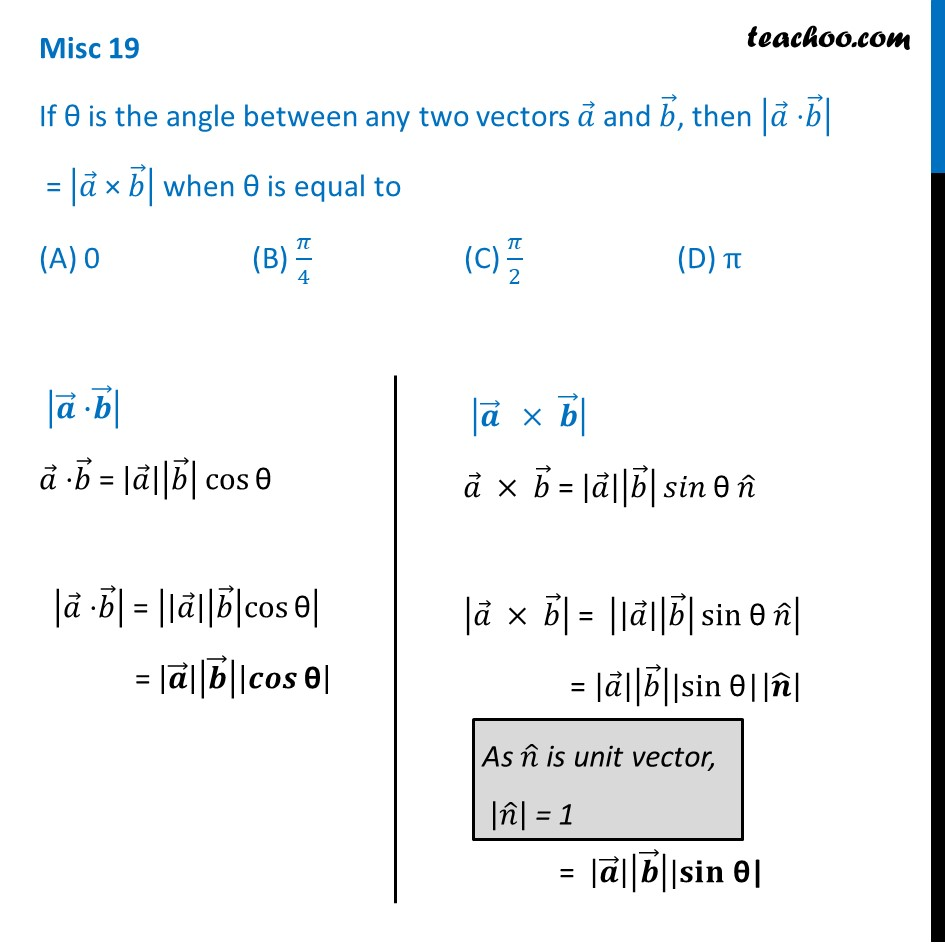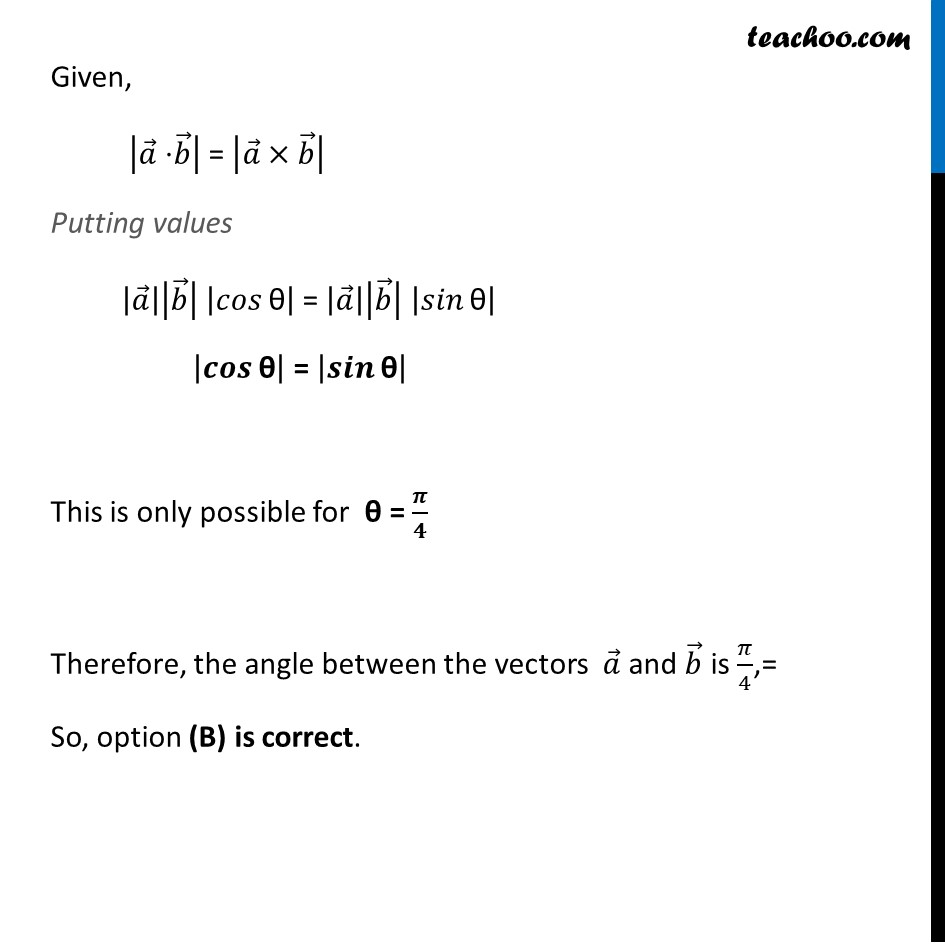Miscellaneous

Chapter 10 Class 12 Vector Algebra
Serial order wiseGet live Maths 1-on-1 Classs - Class 6 to 12

### Transcript

Misc 19 If θ is the angle between any two vectors 𝑎 ⃗ and 𝑏 ⃗, then |𝑎 ⃗" ⋅" 𝑏 ⃗ | = |𝑎 ⃗" × " 𝑏 ⃗ | when θ is eq |𝒂 ⃗" ⋅" 𝒃 ⃗ | 𝑎 ⃗" ⋅" 𝑏 ⃗ = |𝑎 ⃗ ||𝑏 ⃗ | cos⁡"θ" |𝑎 ⃗" ⋅" 𝑏 ⃗ | = ||𝑎 ⃗ ||𝑏 ⃗ |cos⁡"θ" | = |𝒂 ⃗ ||𝒃 ⃗ ||𝒄𝒐𝒔⁡"θ" | |𝒂 ⃗" " ×" " 𝒃 ⃗ | 𝑎 ⃗ × 𝑏 ⃗ = |𝑎 ⃗ ||𝑏 ⃗ | 𝑠𝑖𝑛⁡"θ" 𝑛 ̂ |𝑎 ⃗ × 𝑏 ⃗ | = ||𝑎 ⃗ ||𝑏 ⃗ |⁡〖sin" θ " 𝑛 ̂ 〗 | = |𝑎 ⃗ ||𝑏 ⃗ ||sin" θ|" |𝒏 ̂ | = |𝒂 ⃗ ||𝒃 ⃗ ||𝐬𝐢𝐧" θ|" As 𝑛 ̂ is unit vector, |𝑛 ̂ | = 1 Given, |𝑎 ⃗" ⋅" 𝑏 ⃗ | = |𝑎 ⃗×𝑏 ⃗ | Putting values |𝑎 ⃗ ||𝑏 ⃗ | |𝑐𝑜𝑠⁡"θ" | = |𝑎 ⃗ ||𝑏 ⃗ | |𝑠𝑖𝑛⁡"θ" | |𝒄𝒐𝒔⁡"θ" | = |𝒔𝒊𝒏⁡"θ" | This is only possible for θ = 𝝅/𝟒 Therefore, the angle between the vectors 𝑎 ⃗ and 𝑏 ⃗ is 𝜋/4,= So, option (B) is correct. ual to (A) 0 (B) 𝜋/4 (C) 𝜋/2 (D) π